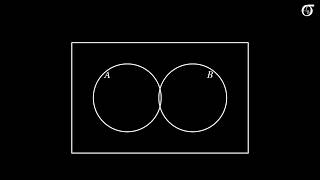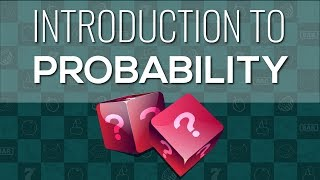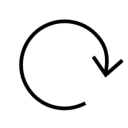complement ⪢⪢
When two angles are complementary, we say that one angle is the _____ of the other. #math
http://qindex.info/i.php?x=9593◎◎◎ complementary ⪢⪢
If two angles are _____ , then the sum of their measures is 90 degrees. ￫ measure #math
http://qindex.info/i.php?x=9684
complement ⪢⪢
#bookmark
http://qindex.info/i.php?x=3796￤ᑅ±ᑀ2020-04-20
Basics of Probability: Unions, Intersections, and Complements
http://qindex.info/i.php?x=9148◌◌◌ probability
What is probability | Expected Values, Frequency Distribution, Complement
http://qindex.info/i.php?x=9150
-----